# Famous Loss Functions and Cost Functions in Machine Learning

Optimization algorithms are the heart of Machine learning algorithms, as most ML algorithms get reduced to optimizing functions. But have we ever thought about what exactly they optimize? In this article, we will try to find the answer to this question.

### Key takeaways from this blog

After going through this blog, we will be familiar with

1. What is the loss function, and why is it important?
2. What are the loss functions used for regression tasks? Absolute loss, Square loss, and Huber loss.
3. What are the loss functions used for binary classification tasks? Binary cross-entropy and Hinge loss.
4. What are the loss functions used for multiple classification tasks? Categorical cross-entropy.

Before moving any further, let's define the term of loss function for us and see one famous optimizer algorithm, gradient descent, and learn how it helps find the optimum values of parameters faster.

#### Loss Function

In the supervised learning approach, whenever we build any machine learning model, we try to minimize the error between the predictions made by our model and the corresponding true values. That error comes from the loss function.

For example, suppose we are designing an email spam classifier model via our machine learning algorithms. In that case, we want to ensure that if any new email comes into our inbox, it must be accurately categorized with spam or non-spam tag. But how do we check that? That’s where the role of loss function comes into the picture.

#### Difference between Loss and Cost Function

We usually consider both terms as synonyms and think that they can be used interchangeably. But, the Loss function is associated with every training example, and the cost function is the average value of the loss function over all the training samples. In Machine learning, we usually try to optimize our cost function rather than loss function.

#### Why is the choice of the perfect loss function important?

There is one famous quote in Neural Smithing Book,

It is important, therefore, that the function faithfully represent our design goals. If we choose a poor error function and obtain unsatisfactory results, the fault is ours for badly specifying the goal of the search.

Loss functions are the translation of our need from machine learning in a mathematical or statistical form. If we know what exactly we want to achieve, it will make the process easier.

#### Optimization algorithm of Gradient Descent

Suppose J(θ) is the loss function and θ is the parameters that the machine learning model will learn. Suppose we are making our loss function continuous functions, and the model wants to learn those parameters for which the loss will be minimal. But how will the model reach those parametric values? That's where we need some optimization algorithm where we need to optimize our cost function.

Gradient descent is quite a famous optimization algorithm in machine learning, so let’s see how it works.

The overall process of the Gradient Descent algorithm,

1. Initialize the weight values randomly.
2. Partially differentiate the cost function G = ∂J(θ)/∂θ w.r.t different parameters constituting the cost function.
3. Update the weights by an amount proportional to gradient to ensure that loss reduces in each iteration, θ = θ-α.G. Here α is the learning rate parameter which is considered a vital hyperparameter. It decides how fast updates should be done.
4. Repeat the process of updation until the change in values of the cost function between two consecutive iterations goes beyond the threshold.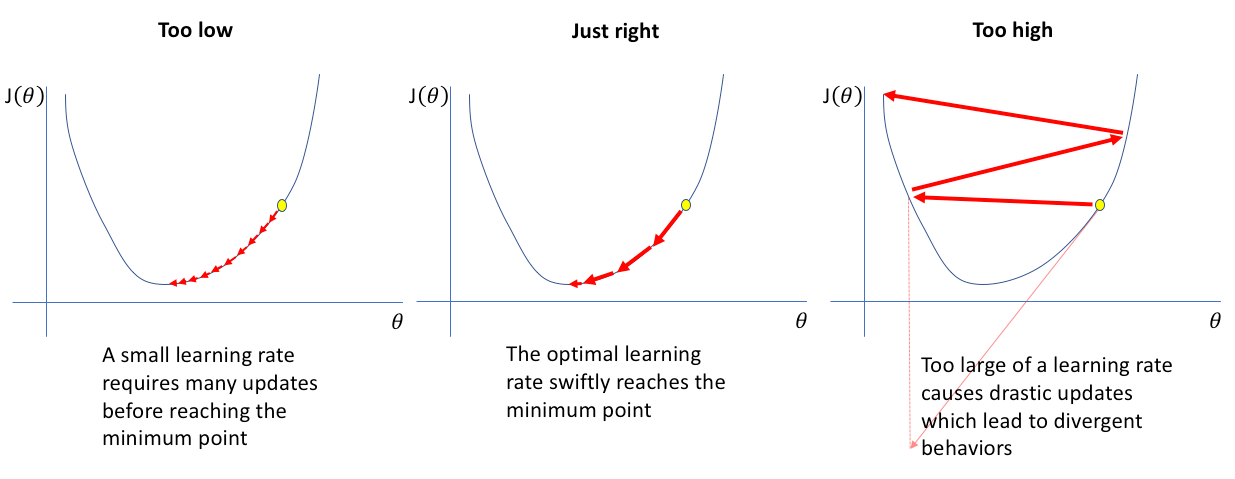Based on the nature of the problem statement, we categorize machine learning models into three classes,

• Classification
• Regression
• Clustering

But clustering is not a supervised learning approach. So we have two cases left, Regression and Classification problem statements. Some widespread and readymade loss functions are available in these categories, and now we will discuss that.

### Regression Loss Functions

In regression tasks, we try to predict the continuous target variables. Suppose we are trying to fit the function f using machine learning on the training data X = [X1, X2,…, Xn] so that f(x) fits Y = [Y1, Y2,…, Yn]. But this function “f” can not be perfect, and there will be errors in the fitting.

#### Square Error

This is also called L2 loss. If we observe the loss function, it’s a quadratic equation that only has a global minimum and no local minima. We can say that it has a mathematical advantage. This makes it one of the most favorable loss functions among data scientists and machine learning professionals.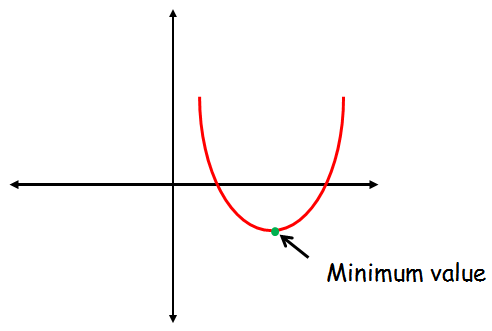The corresponding cost function will be the average of these losses for all the data samples, also called Mean Squared Error (MSE).Using gradient descent here and looking onto the update term, θ = θ-α.G, we can say that “higher the error, the more changes will happen in the parameter”. Or we can also say, more penalization will happen when the error is higher. But what if there are outliers in the training sample?

The difference will be higher, and after squaring that, it will make them even more prominent. So MSE is less robust to outlier presence and hence advised to not use MSE as the loss function when there are too many outliers present in the dataset.

#### Absolute Error

This is also called L1 loss. Unlike MSE, here, we take the absolute value of the error rather than squaring it. But there is a drawback. Finding gradients involve more complicated linear programming techniques. It is also widely used in industries, especially when the training data is more prone to outliers.The corresponding cost function will be the average of the absolute losses over training samples, also called Mean Absolute Error (MAE).#### Huber Loss

L1 and L2 losses are prevalent, but there are limitations associated with them,

• L1 loss is more robust to outliers than L2, or we can say that when the difference is higher, L1 is more stable than L2.
• L2 loss is more stable than the L1 loss, especially when the difference between prediction and actual is smaller.

Huber loss takes the good from both L1 and L2 and avoids their shortcomings. It is quadratic for smaller errors and becomes linear for higher values of errors.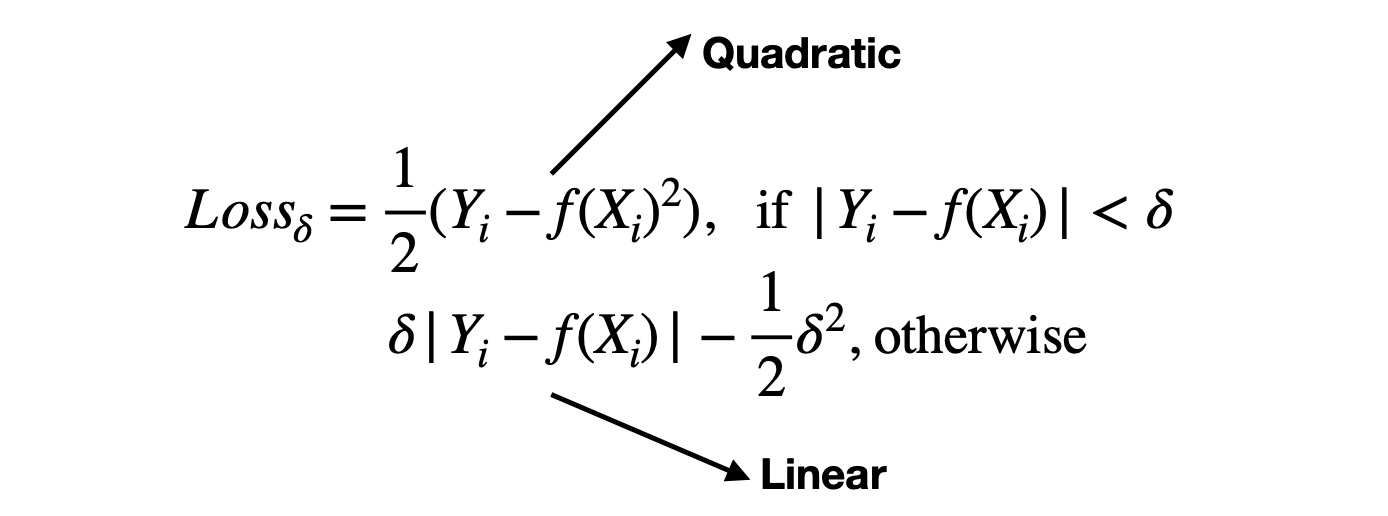Huber loss function is characterized by the parameter δ.

### Classification Loss Functions

We try to predict the categorical values instead of continuous ones for the target variables in classification tasks. For example, suppose we want to classify the incoming emails as spam or non-spam. Target variables are categorical here.

To predict the categorical variables, we take the help of probability theory. Suppose our machine learning classification model is saying that email is spam with a probability of 0.9. In that case, we can say that model is 90% confident, so this mail should be categorized as spam, and its true value was also spam. In such a case, the error will be zero. But if we somehow misclassify it, then the error value will be 1. But with this definition of error, we will never be able to find the gradients and suitable parameters for that.

To tackle this situation, we treat the predicted probabilities as the samples coming from one probability density function and actual probabilities coming from another probability density function. And the objective is to match these PDFs.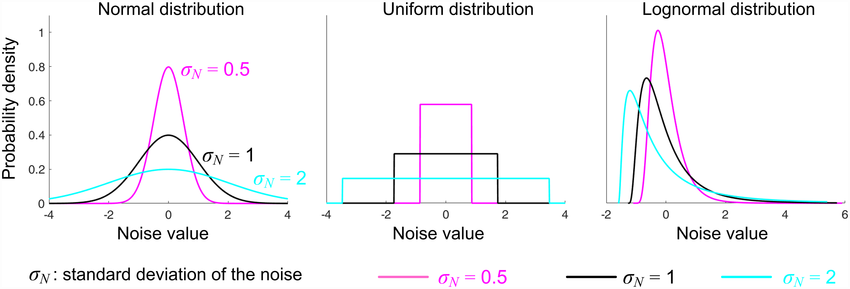Source: Researchgate

Before going any further, let’s understand the term entropy first. Entropy signifies uncertainty. For a random variable X, having probability distribution as p(X), entropy is defined as: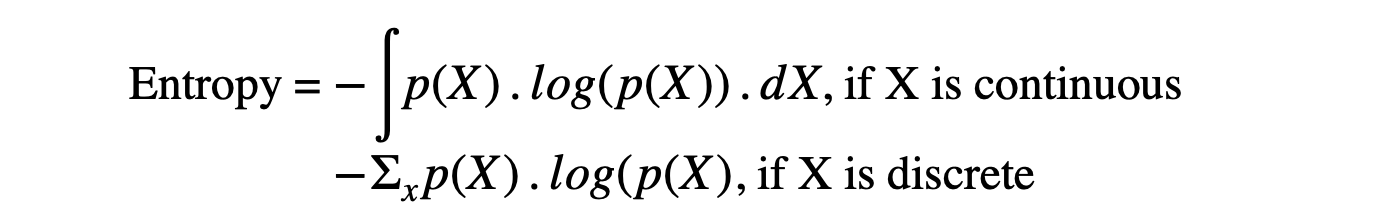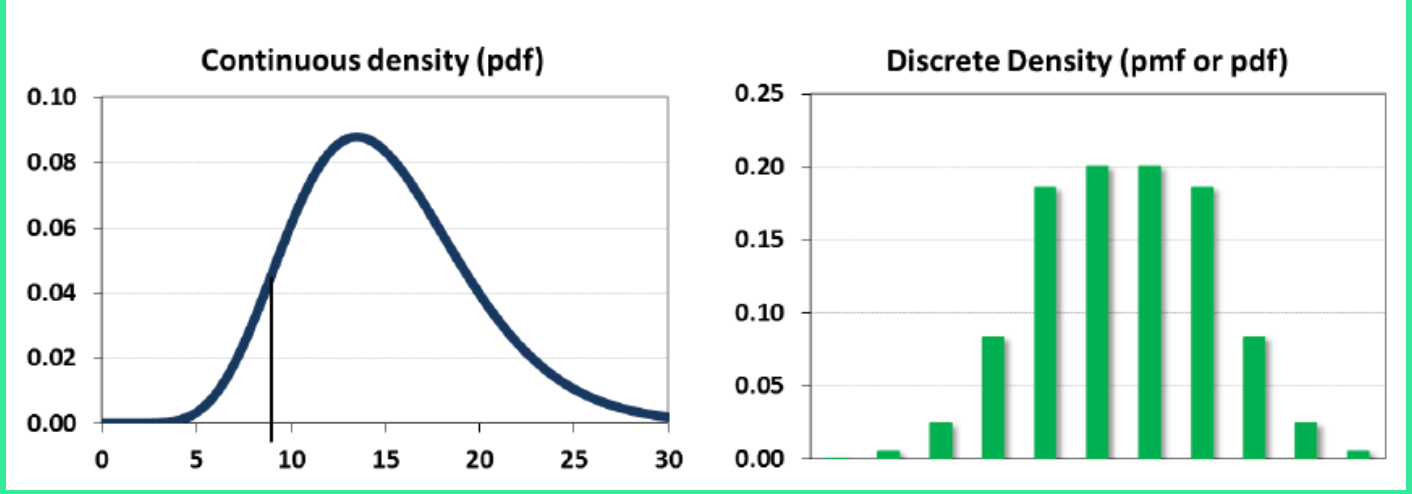Source: Brainstudy.info

If the entropy is higher, the surety of the distribution function will be lesser, and when the entropy is lower, the confidence or surety will be higher.

#### Binary Cross-Entropy

Here we have the target variables in the binary format, or we can say that only two classes are present. If the probability of being in class 1 is P, then the probability of being in class 2 will be (1-P).

Cross entropy loss for the actual label of Y (which can take values either 0 or 1) and the predicted probability of P can be defined as,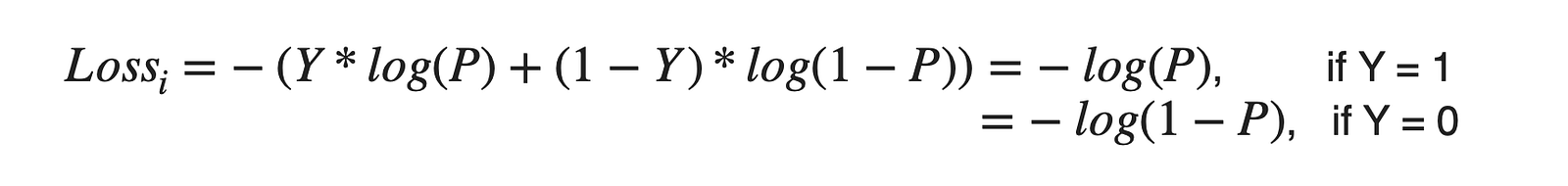This loss is also known as the Log loss. We can use the sigmoid function to calculate P, where Z represents the input parameters to the model.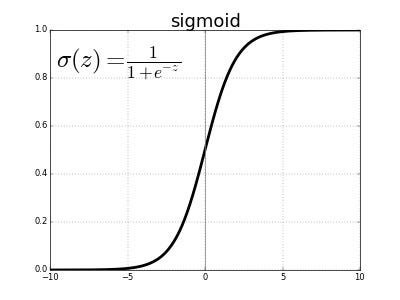The corresponding cost function will be defined as,#### Categorical Cross-Entropy

Here we have the categorical variables in the form of multiple classes. The entropy calculation will remain the same, but the Y vector will be represented as the One-hot encoded vector. Suppose there are three classes of Cat, Dog, and nocatno_dog. One-hot representation of these classes can be,

Cat = [1,0,0], Dog = [0,1,0], nocatno_dog = [0,0,1].C = total classes

So, Yij will be in one-hot vector representation form, and Pij will be the predicted probability for being in class j when the i-th sample of input (Xi) is provided. Unlike binary cases, here, we use the softmax function to calculate Pij.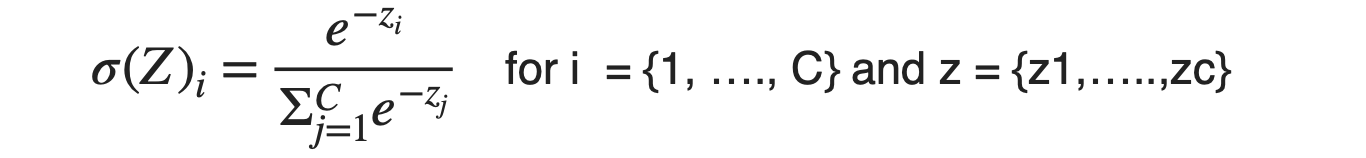Note: The sum of all these values of σi will be 1 as the denominator, and the numerator will become identical.

#### Hinge Loss

This special loss function is only used with Support Vector Machines or Maximal Margin Classifiers, having classes -1 and 1 ( Not 0 and 1). SVM is a machine learning algorithm specially used for binary classification and uses decision boundaries to separate two classes.

Hinge loss penalizes the wrong predictions as well as the predictions for which model is less confident.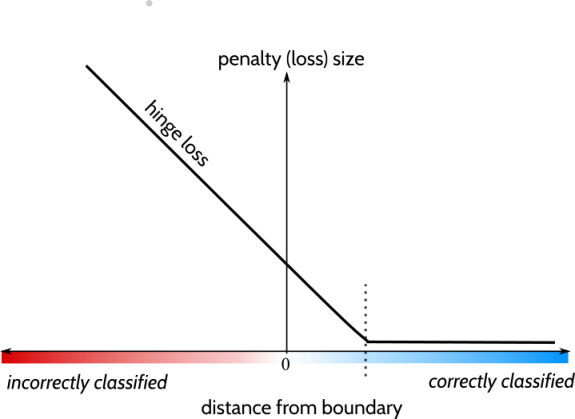It is a non-differentiable function but possesses convex nature, which helps in finding the optimal loss.

### Possible Interview Questions

This is one of the most frequently asked topics in machine learning interviews. Interviewers mainly focus on checking the understanding of how ML algorithms work. Some frequent questions are:

1. What are the different loss functions you tried for your regression model, and why did you finalize the final one?
2. What makes Support Vector Machine different from rest of the machine learning algorithms?
3. What is the difference between binary cross-entropy and categorical cross-entropy?
4. What is the difference between cost function and loss function?
5. If Huber loss is better, why do we generally see MSE and MAE as cost functions?

### Conclusion

In this article, we learned several loss functions which are highly popular in the machine learning domain. We learned different loss functions used in Classification as well as regression tasks. In the regression problem statement, we learned square loss, absolute loss, and the Huber loss. While in classification tasks, we learned binary cross-entropy, categorical cross-entropy, and the special hinge loss used in maximal margin classifiers. We hope this has cleared all your confusion about when to choose which loss function.

#### Enjoy Learning! Enjoy Thinking! Enjoy Algorithms!

Get well-designed application and interview centirc content on ds-algorithms, machine learning, system design and oops. Content will be delivered weekly.